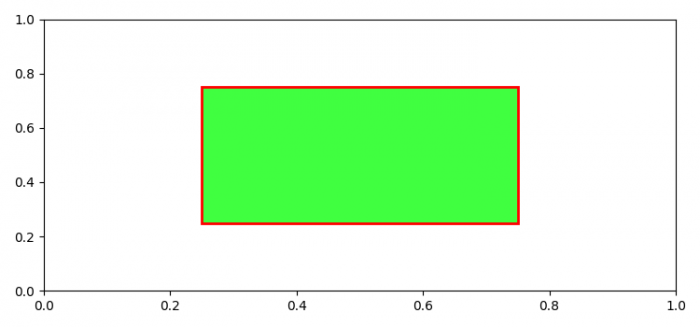# How to set different opacity of edgecolor and facecolor of a patch in Matplotlib?

To set different opacity of edge and face color, we can use a color tuple and the 4th index of the tuple could set the opacity value of the colors.

## Steps

• Set the figure size and adjust the padding between and around the subplots.
• Create a figure and a set of subplots using subplots() method.
• Set different values for edge and face color opacity.
• To display the figure, use show() method.

## Example

from matplotlib import pyplot as plt, patches

plt.rcParams["figure.figsize"] = [7.50, 3.50]
plt.rcParams["figure.autolayout"] = True

figure, ax = plt.subplots()
edge_color_opacity = 1 # 0<val<1
face_color_opacity = 0.75 # 0<val<1

plt.show()